# Solution Assignment 01 Regression analysis

The data for this assignment are listed in the Excel file Quadratic fuel model.
Car designers have been experimenting with ways to improve gas mileage for many years. An important element in this research is the way in which a car’s speed affects how quickly fuel is burned. Competitions with the objective of driving the farthest on the smallest amount of gas have determined that low speeds and high speeds are inefficient. Designers would like to know which speed burns gas most efficiently. As an experiment, 50 identical cars are driven at different speeds and the gas mileage measured. They use the following model which may describe the relation between gas mileage and speed:

Mileage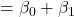speedspeed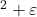a. Explain why the designers assumed that this model may be correct. Also, predict the sign of the coefficient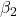without doing any computer computations.
b. Perform a regression analysis using your model and give the regression equation.
c. How well does it fit? Consider the coefficient of determination and the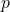-value of the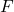-test.
d. Test the coefficients. What may you conclude from this?
e. If you accept this model, which speed would optimize the mileage and why?

Solution.
a. The assumption is that mileage is lowest for low and high speeds, so higher for medium speeds. Such a model can be described by a parabola that ‘opens down’ and has an optimum. In that case.
b. The regression analysis results in the model:
Mileage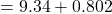speed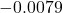speedc. The model fits moderately well. The coefficient of determination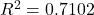is relatively high and the-value ofequals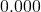.
d. From the-tests for speed and speedyou may conclude that both are linearly related to mileage because of their very low-values.
e. The model is quadratic and the graph opens up. Then the optimal speed is expected to be speed=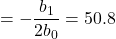miles/hr.